# APPLICATIONS OF TRIGONOMETRIC FUNCTIONS When solving a right

• Slides: 16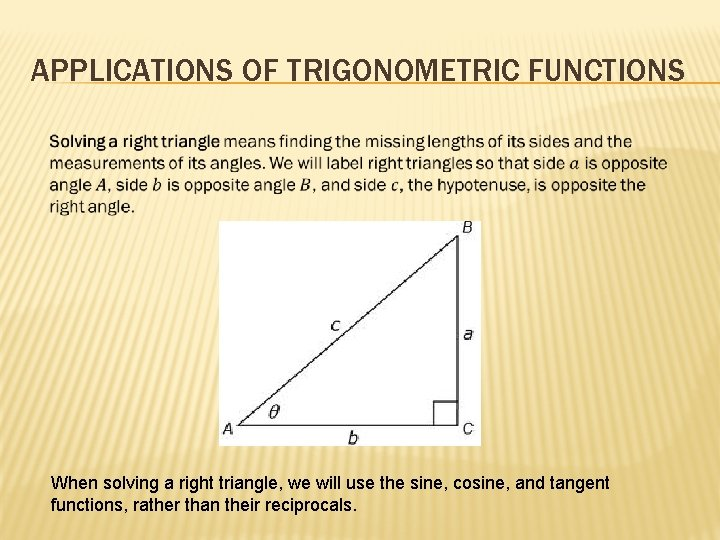APPLICATIONS OF TRIGONOMETRIC FUNCTIONS When solving a right triangle, we will use the sine, cosine, and tangent functions, rather than their reciprocals.SOLVING RIGHT TRIANGLES Solve the right triangle shown below, rounding lengths to two decimal places.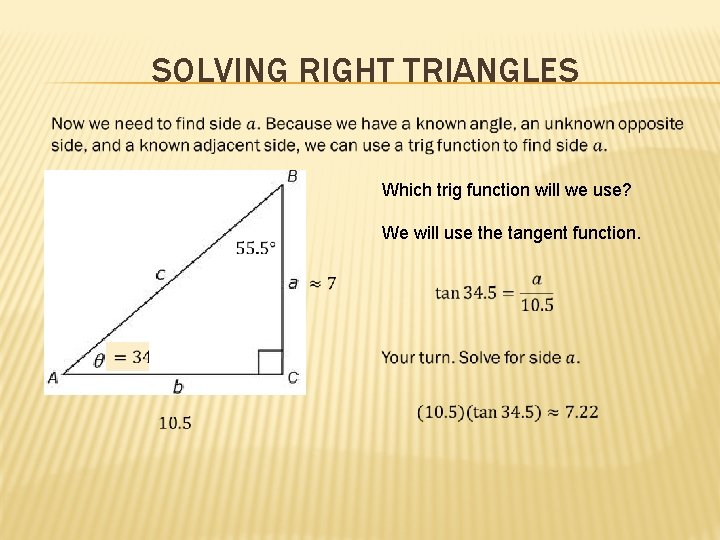SOLVING RIGHT TRIANGLES Which trig function will we use? We will use the tangent function.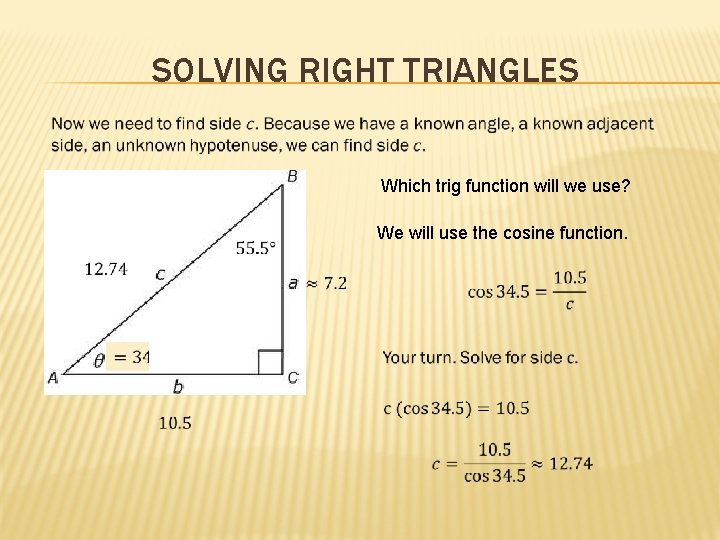SOLVING RIGHT TRIANGLES Which trig function will we use? We will use the cosine function.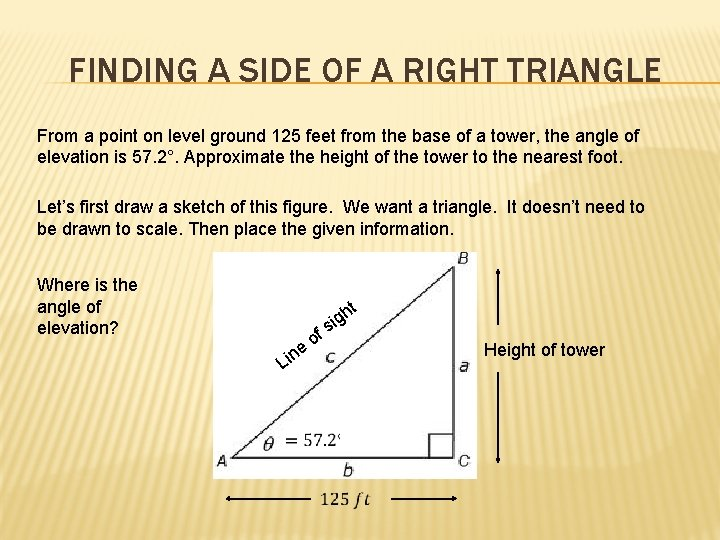FINDING A SIDE OF A RIGHT TRIANGLE From a point on level ground 125 feet from the base of a tower, the angle of elevation is 57. 2°. Approximate the height of the tower to the nearest foot. Let’s first draw a sketch of this figure. We want a triangle. It doesn’t need to be drawn to scale. Then place the given information. Where is the angle of elevation? f ht g i s o e Lin Height of towerFINDING A SIDE OF A RIGHT TRIANGLE Which trig function will we use to find the height of the tower? f ht g i s o e Lin tangent The height of the tower is approximatel y 194 ft. Height of tower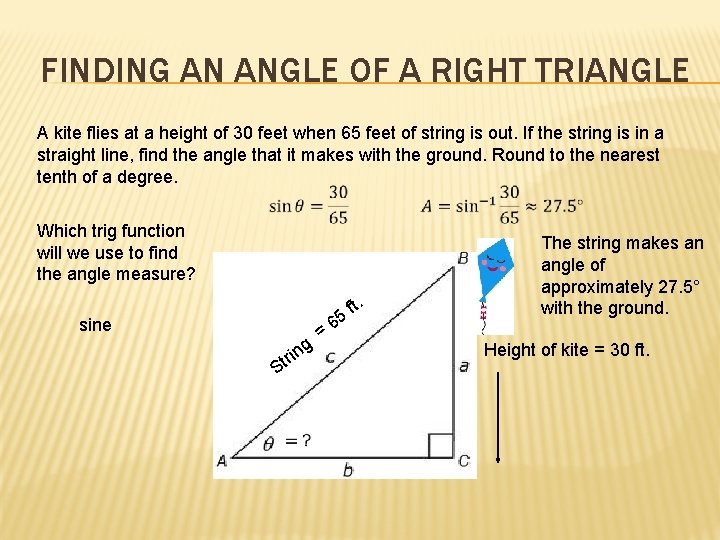FINDING AN ANGLE OF A RIGHT TRIANGLE A kite flies at a height of 30 feet when 65 feet of string is out. If the string is in a straight line, find the angle that it makes with the ground. Round to the nearest tenth of a degree. Which trig function will we use to find the angle measure? . sine = g n ri St ft 5 6 The string makes an angle of approximately 27. 5° with the ground. Height of kite = 30 ft.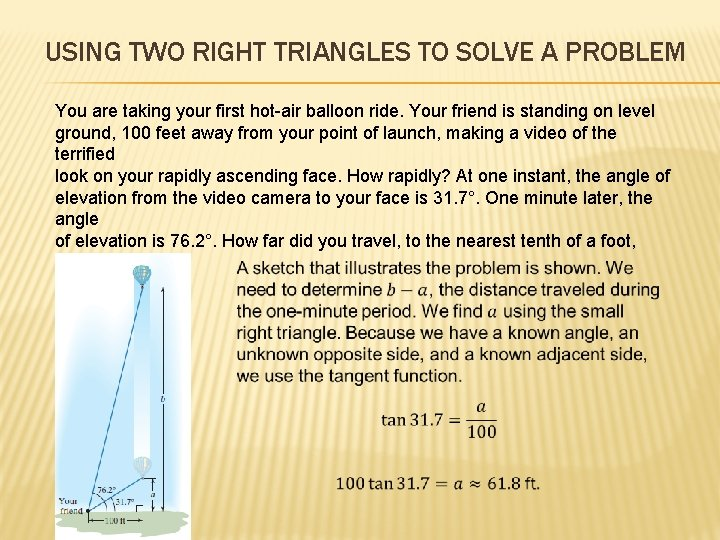USING TWO RIGHT TRIANGLES TO SOLVE A PROBLEM You are taking your first hot-air balloon ride. Your friend is standing on level ground, 100 feet away from your point of launch, making a video of the terrified look on your rapidly ascending face. How rapidly? At one instant, the angle of elevation from the video camera to your face is 31. 7°. One minute later, the angle of elevation is 76. 2°. How far did you travel, to the nearest tenth of a foot, during that minute?USING TWO RIGHT TRIANGLES TO SOLVE A PROBLEMTRIGONOMETRY & BEARINGS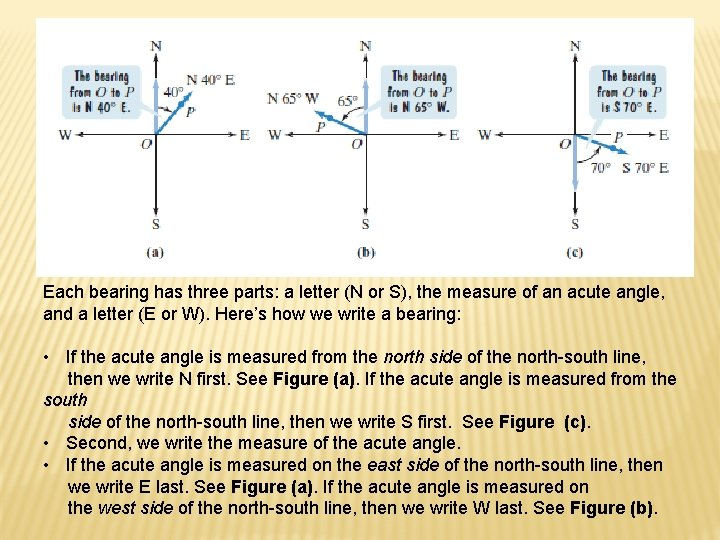Each bearing has three parts: a letter (N or S), the measure of an acute angle, and a letter (E or W). Here’s how we write a bearing: • If the acute angle is measured from the north side of the north-south line, then we write N first. See Figure (a). If the acute angle is measured from the south side of the north-south line, then we write S first. See Figure (c). • Second, we write the measure of the acute angle. • If the acute angle is measured on the east side of the north-south line, then we write E last. See Figure (a). If the acute angle is measured on the west side of the north-south line, then we write W last. See Figure (b).UNDERSTANDING BEARINGS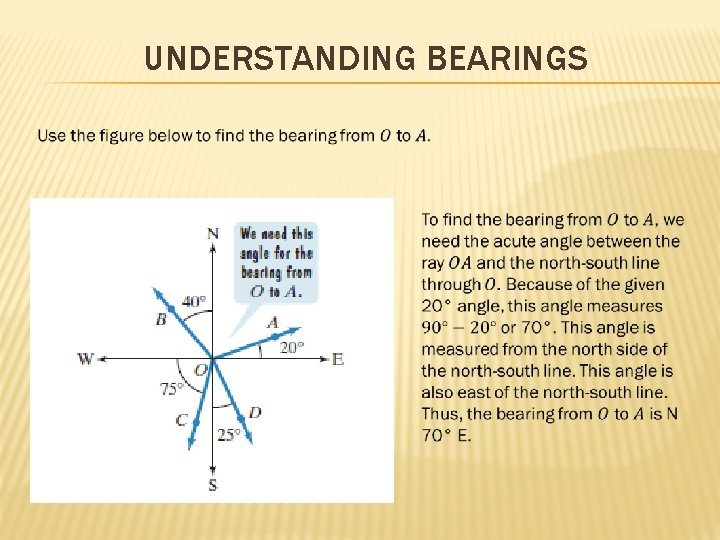UNDERSTANDING BEARINGS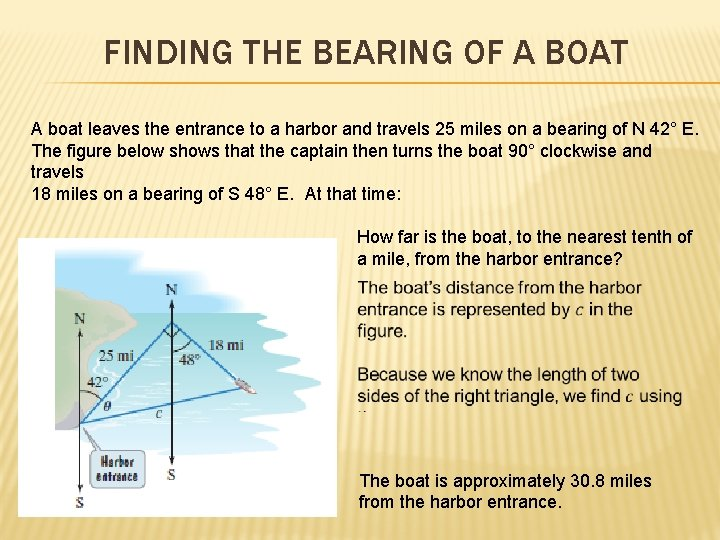FINDING THE BEARING OF A BOAT A boat leaves the entrance to a harbor and travels 25 miles on a bearing of N 42° E. The figure below shows that the captain then turns the boat 90° clockwise and travels 18 miles on a bearing of S 48° E. At that time: How far is the boat, to the nearest tenth of a mile, from the harbor entrance? The boat is approximately 30. 8 miles from the harbor entrance.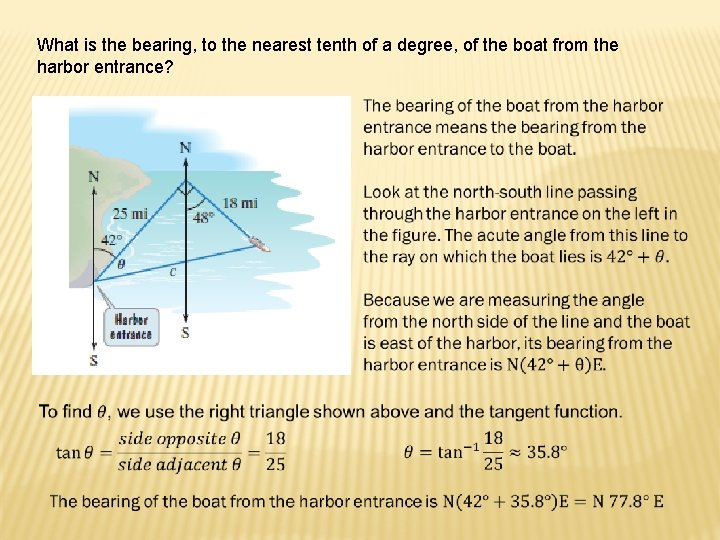What is the bearing, to the nearest tenth of a degree, of the boat from the harbor entrance?